# 4. Determine the vertical displacement of point C using Castigliano's theorem. Include axial displacements. Assume E,...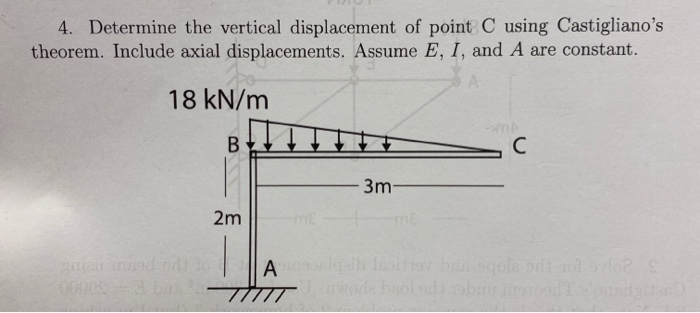4. Determine the vertical displacement of point C using Castigliano's theorem. Include axial displacements. Assume E, I, and A are constant. 18 kN/m 3m- 2m Alle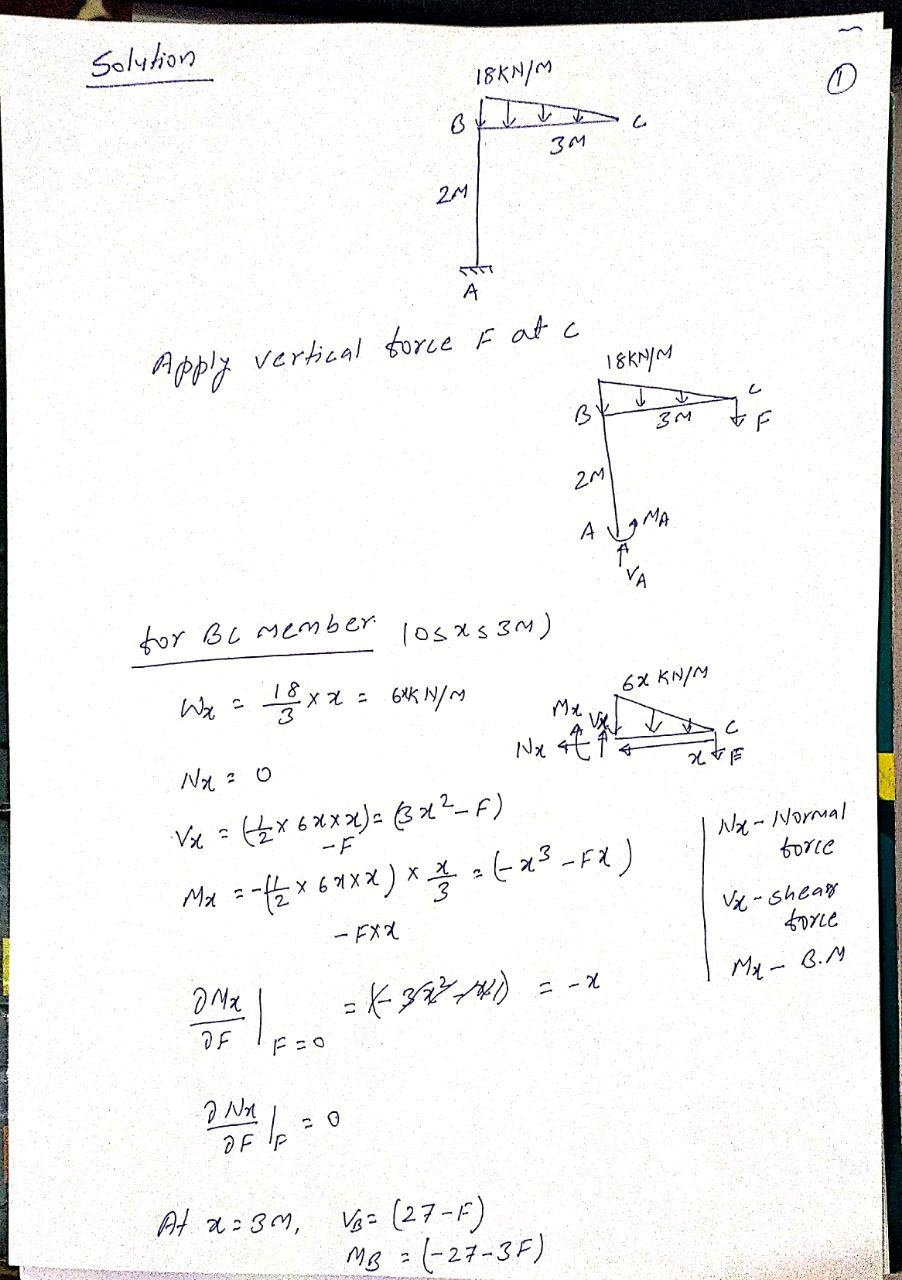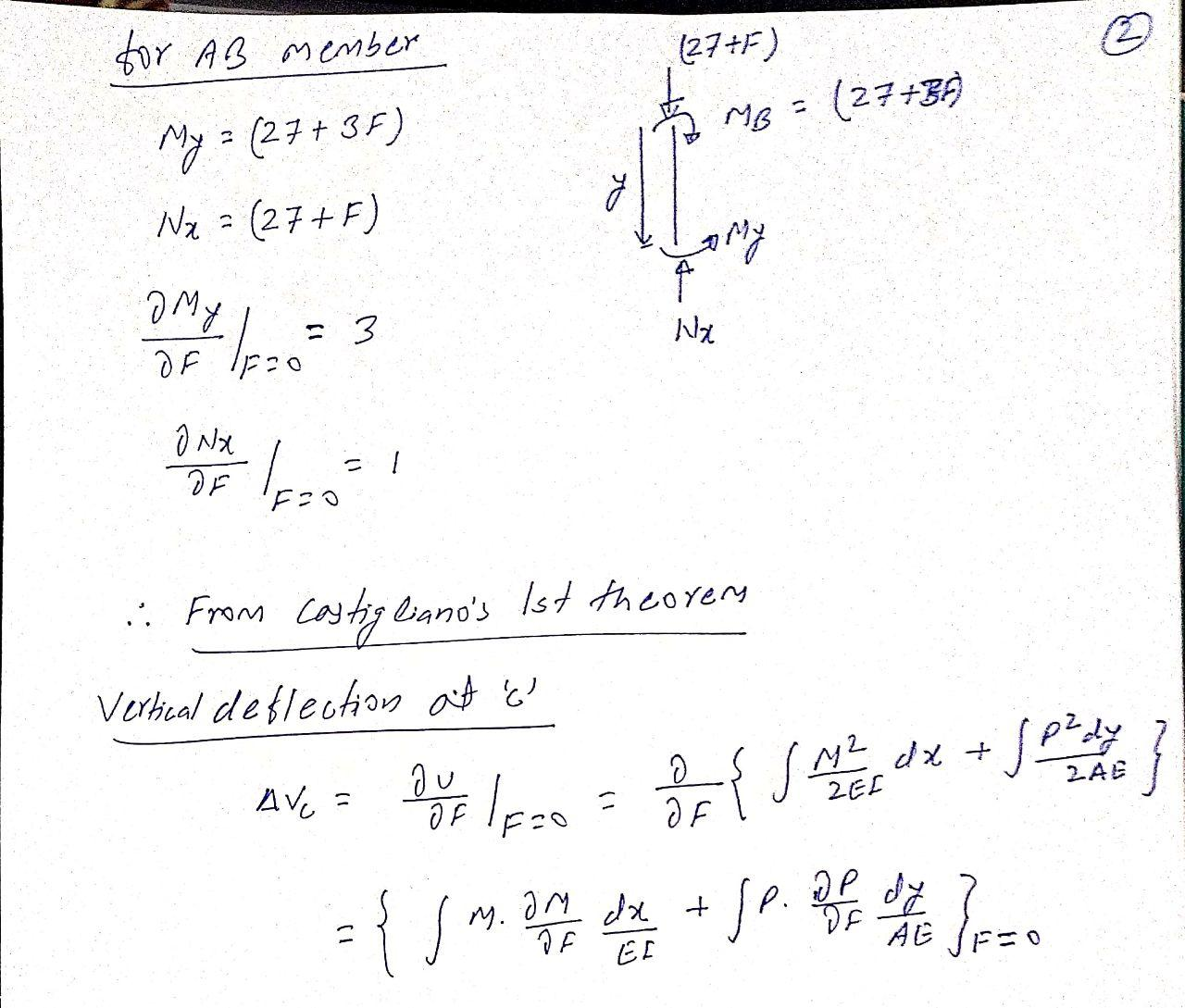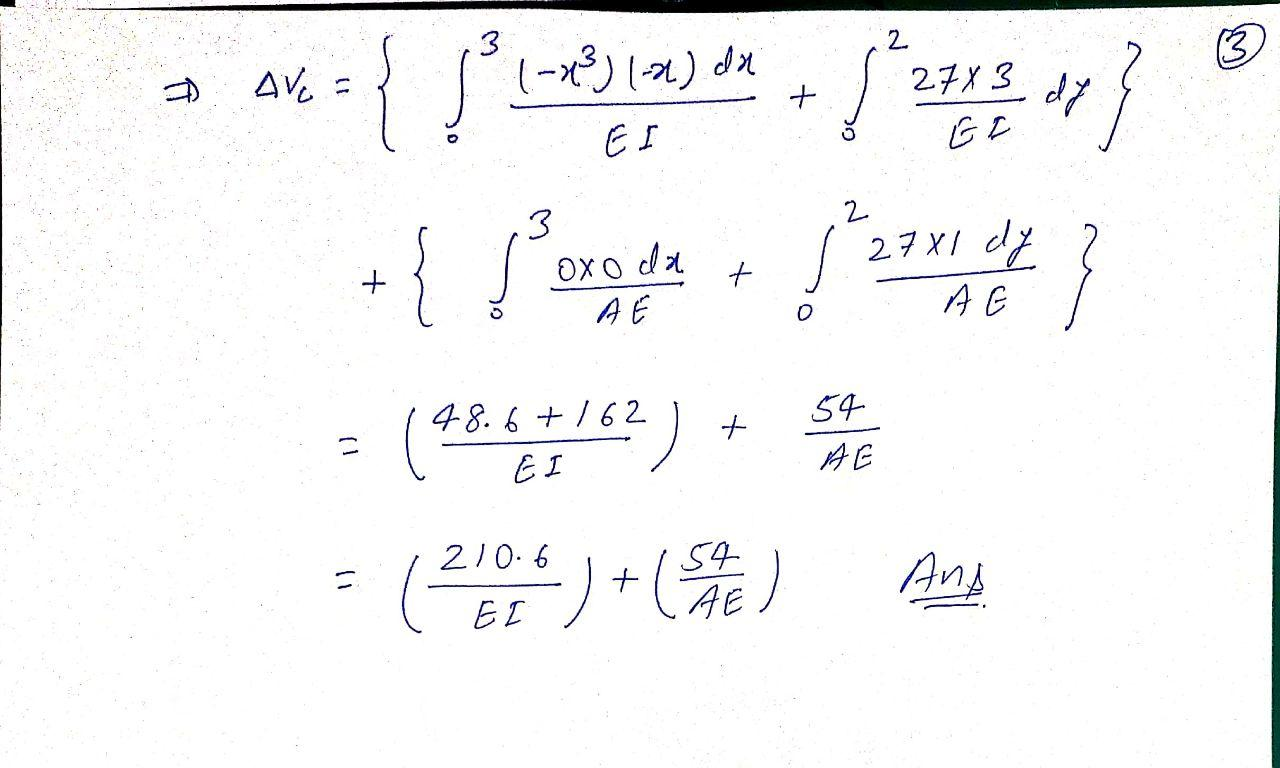#### Earn Coin

Coins can be redeemed for fabulous gifts.

Similar Homework Help Questions
• ### 2. Determine the vertical displacement at joint B and horizontal displacement at joint D using Castigliano's...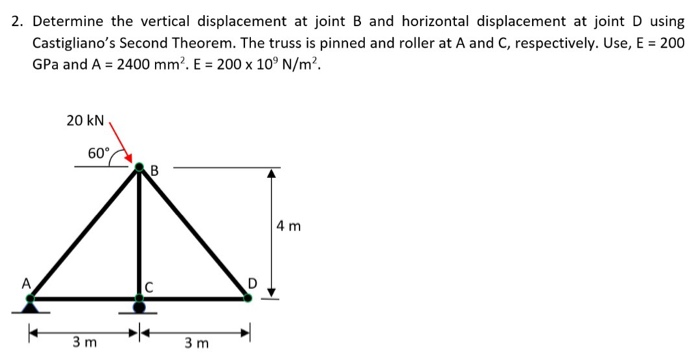2. Determine the vertical displacement at joint B and horizontal displacement at joint D using Castigliano's Second Theorem. The truss is pinned and roller at A and C, respectively. Use, E = 200 GPa and A = 2400 mm. E = 200 x 10°N/m². 20 KN 60 CAB 4 m 3m 3m

• ### 8-55 : Determine the vertical displacement of point C. EI is constant. Use the method of...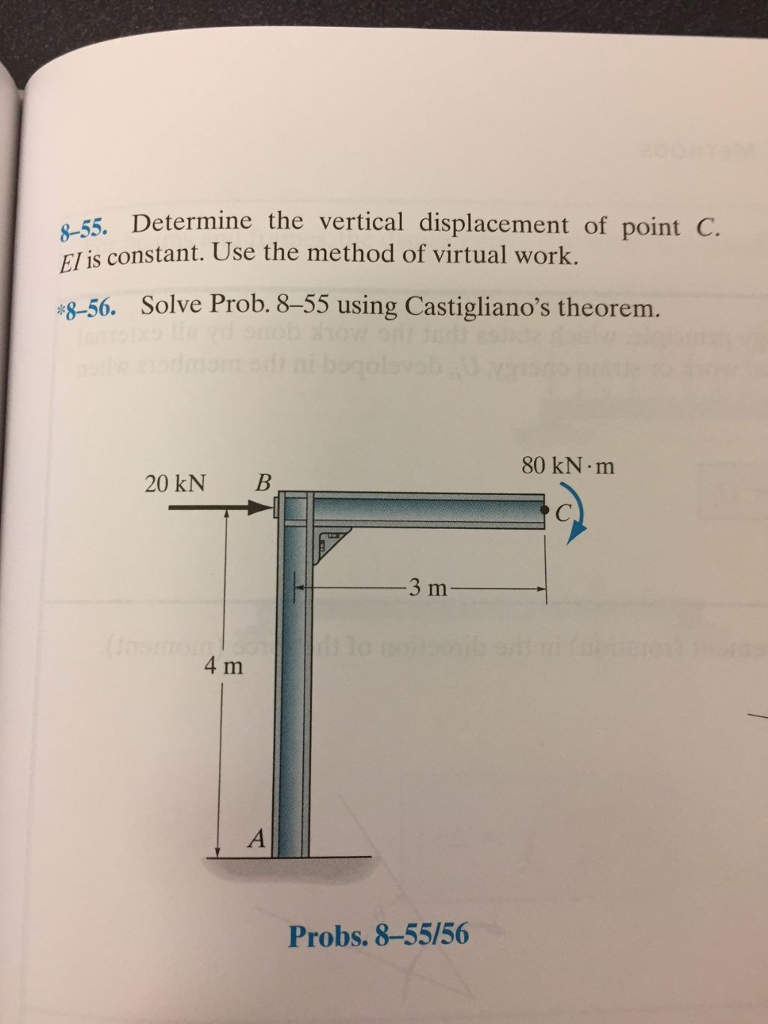8-55 : Determine the vertical displacement of point C. EI is constant. Use the method of virtual work. -55. Determine the vertical displacement of point C. El is constant. Use the method of virtual work. \$-56. Solve Prob. 8-55 using Castigliano's theorem. 80 kN m 20 kN B 4 m Probs. 8-55/56

• ### Problem 4 Write the total strain energy and use Castigliano's theorem to determine the vertical displacement...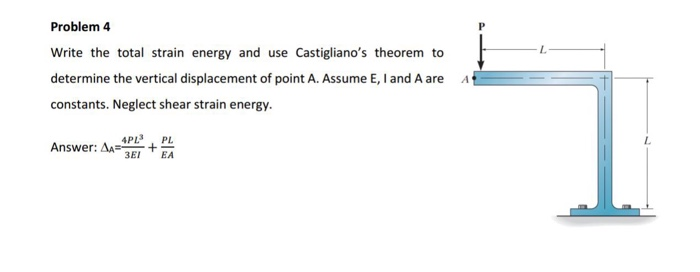Problem 4 Write the total strain energy and use Castigliano's theorem to determine the vertical displacement of point A. Assume E, I and A are A constants. Neglect shear strain energy. L. Answer: ?- + E1 EA

• ### * J Determine the slope at A and displacement at point C. El is constant. Use...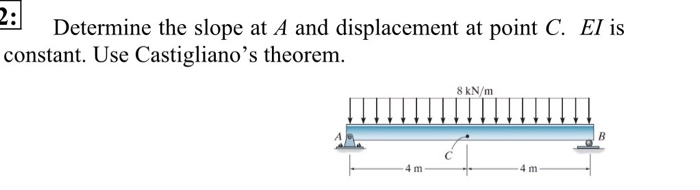* J Determine the slope at A and displacement at point C. El is constant. Use Castigliano's theorem. 8 kN/m - 4 m 3: Determine the displacement at point C. EI is constant. Use Castigliano's theorem. (Page 1 of 1 Determine the slope and displacement at point A. El is constant. Use Castigliano's theorem. ON

• ### SOLVE B AND C USING CASTIGLIANO'S THEOREM Consider the cantilever beam shown in . Suppose that...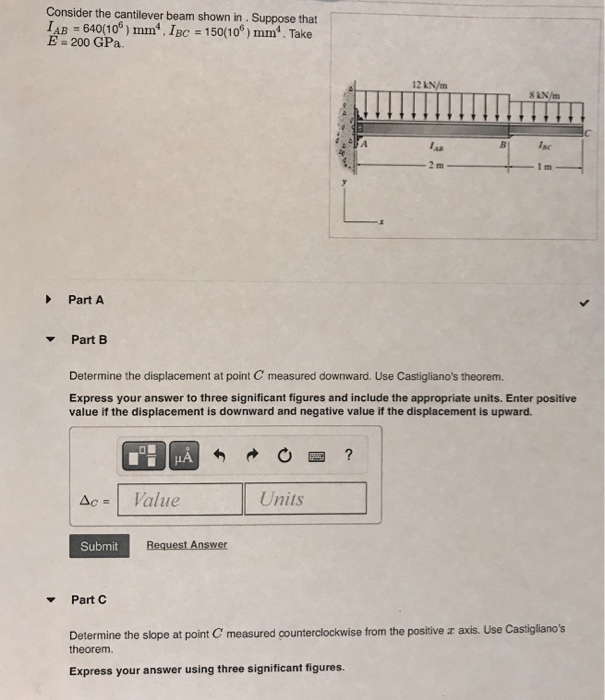SOLVE B AND C USING CASTIGLIANO'S THEOREM Consider the cantilever beam shown in . Suppose that LAB = 640(106) mm IBC = 150(106) mm". Take E 200 GPa 12 kN/m 8 kN/m موا Inc Part A Part B Determine the displacement at point C measured downward. Use Castigliano's theorem. Express your answer to three significant figures and include the appropriate units. Enter positive value if the displacement is downward and negative value if the displacement is upward. HA ? Ac...

• ### Please show all work 4 kN 2. Use Castigliano's theorem to find the vertical displacement of...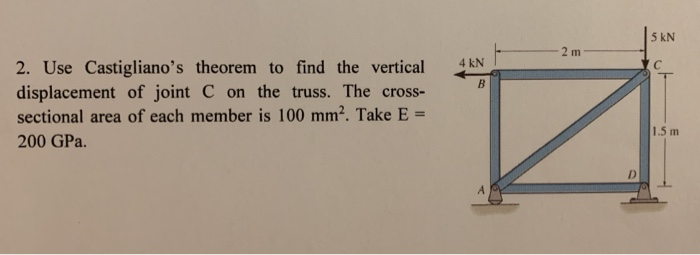Please show all work 4 kN 2. Use Castigliano's theorem to find the vertical displacement of joint C on the truss. The cross- sectional area of each member is 100 mm2. Take E = 200 GPa. 1.5 m

• ### Determine the slope and displacement at point A. Assume C is pinned. (Answer encircled letter only)...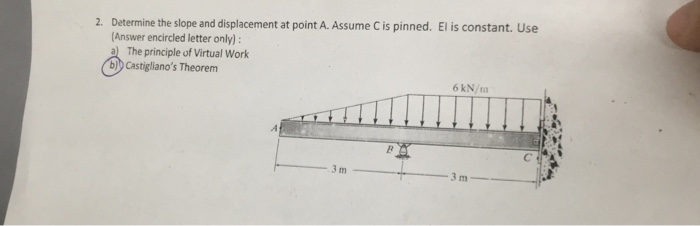Determine the slope and displacement at point A. Assume C is pinned. (Answer encircled letter only) a The principle of Virtual Work b)b Castigliano's Theorem El is constant. Use 2. 6 kN/m 3 m

• ### Use Castigliano's theorem and determine the vertical deflection at C. Include the effects of...

The bent road has an E=200 GPa, G=75 GPa, and a radius of 30 mm. Use Castigliano's theorem and determine the vertical deflection at C. Include the effects of bending,shear, and torsional strain energy.

• ### Castigliano’s Theorem Determine the horizontal and vertical displacement at point E (in millimeters). E = 200...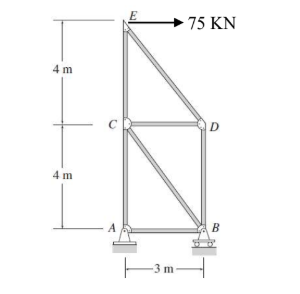Castigliano’s Theorem Determine the horizontal and vertical displacement at point E (in millimeters). E = 200 GPa. All the bars have an area of 5000 mm2 75 KN 4 m 4 m ITm

• ### Use Castigliano's theories to obtain vertical displacement of the truss point C. E 3 m B C A 3 m 3 m 3 m 20 kN 20...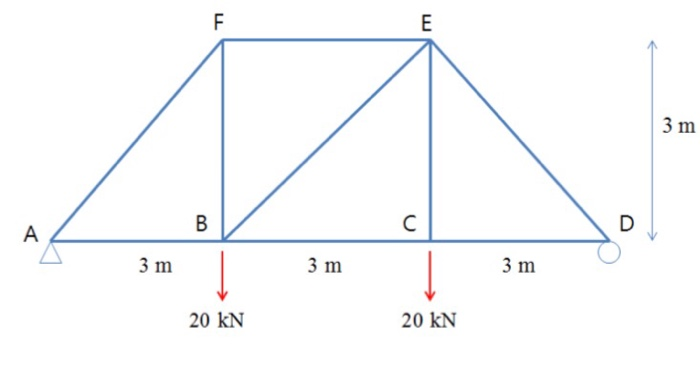Use Castigliano's theories to obtain vertical displacement of the truss point C. E 3 m B C A 3 m 3 m 3 m 20 kN 20 kN LLI LL E 3 m B C A 3 m 3 m 3 m 20 kN 20 kN LLI LL